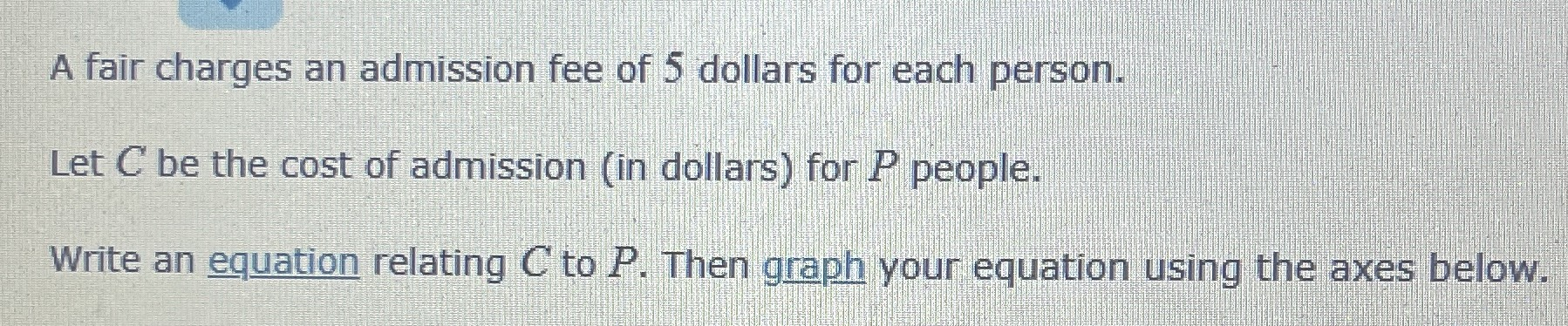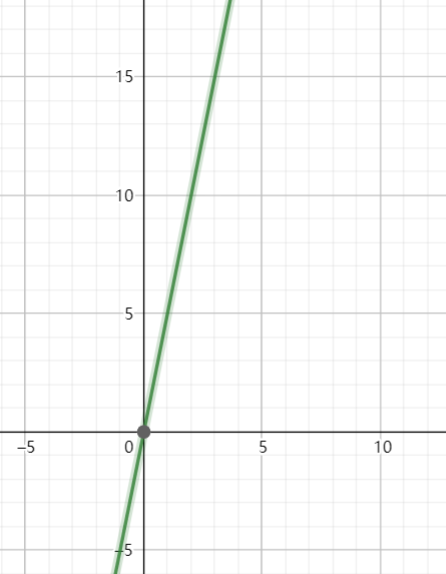### ¿Todavía tienes preguntas de matemáticas?

Pregunte a nuestros tutores expertos
Algebra
PreguntaA fair charges an admission fee of $$5$$ dollars for each person.

Let $$C$$ be the cost of admission (in dollars) for $$P$$ people.

Write an equation relating $$C$$ to $$P$$ . Then graph your equation using the axes below.